Digits and Place Value: Part 2

 Problem Set 1 Problem Set 2 Problem Set 3Select a problem set using the buttons above, then use your mouse or tab key to select a question. Fill in the blank with the correct answer for each problem. When you have answered all of the questions, ask Charlie how you did.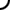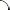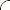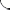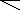Write each number in expanded form. Be sure to use commas where appropriate.   30,208 240,015 7,001,090   Write each word statement using digits. Be sure to use commas where appropriate.   Thirty five million, two hundred fifty seven thousand, twenty one. Two hundred billion, ninety one million, four hundred. Six trillion, eight hundred five billion, twenty million, four thousand, sixteen.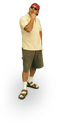Write each number in expanded form. Be sure to use commas where appropriate.   52,041 108,560 72,050,100   Write each word statement using digits. Be sure to use commas where appropriate.   One hundred two million, eighty nine thousand, five hundred twenty. Five billion, six hundred ninety three million, seven hundred fourty one. Four hundred trillion, six hundred ten million, ninety thousand.Write each number in expanded form. Be sure to use commas where appropriate.   20,340 183,400 300,260,005   Write each word statement using digits. Be sure to use commas where appropriate.   Eight million, nine hundred twelve thousand, five hundred two. Thirteen billion, six hundred seven million, sixty. Fifteen trillion, three hundred billion, twenty seven thousand, ten.©  2009  LarryPerezWeb Design:  Patrick Quigley